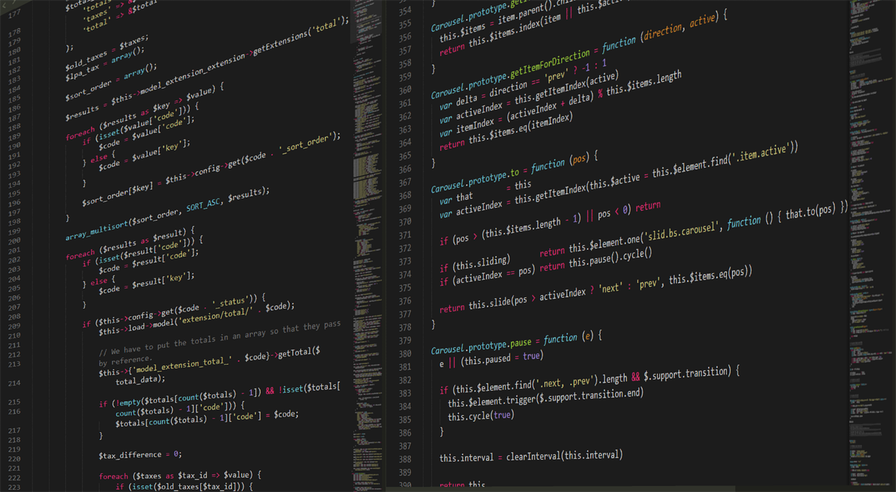# Groovy: What happens when you assign a BigInteger to an IntegerThis question came in from a student in my Apache Groovy Development Course. It was actually quite interesting because it tripped me up at first before I understand what was going on behind the scenes.The student was asking why when they created an integer but assigned to large of a number to it did the results end up like this.

``````int i = 2356524235623432414235234234
println i.class // java.lang.Integer
println i // 1413517242
``````

## Groovy Integer

Looking at the code example above can you see what is going on and why? Let's take a minute and break this down. First, we are setting a very large number (BigInteger) to a data type integer. If you look at the Integer class there is a static MAX_VALUE that is set to "A constant holding the maximum value an  `int`  can have, 231-1."

``````int i = 2356524235623432414235234234
``````

So we are trying to set a value that is outside of that max value range to an integer. When we print out the class though it's still an integer

``````println i.class // java.lang.Integer
``````

When we print out the value though it appears it is some random number.

``````println i // 1413517242
``````

You might expect this to just error out but that isn't the case. What happens underneath the hood is that the number on the right is actually a BigInteger and when you try to assign it to an integer something happens. We end up calling the BigInteger's class method int value which will convert that number to an integer.

``````BigDecimal bd = 2356524235623432414235234234;
println bd.intValue() // 1413517242
``````

## Conclusion

When you understand what is happening under the hood everything seems to make a lot more sense.